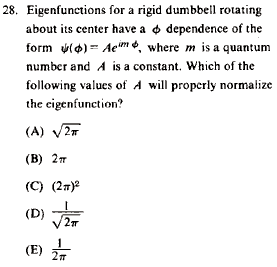GR 8677927796770177 | # Login | Register

GR8677 #28
Problem
 GREPhysics.NET Official Solution Alternate SolutionsQuantum Mechanics}Normalization

Recall that is the condition for a normalized function.

where the condition is used.

This is choice (D).Alternate Solutions
 Giubenez2014-10-22 02:42:06 The only way to solve this problem is to remember the first Spherical Harmonics, or, at least, their dependence to . In fact, using the conventional coordinates where is the polar angle and is the azimutal angle, they always have a term . The direction is to estimate A, we have thus to integrate on that in the question is CAPITALIZED and we have to choose the intervalReply to this commentfredluis
2019-08-09 04:33:09
This problem is deceptively simple, people. Remember that if the charge is moving towards the wire its velocity vector would be negative. tree removaljoshuaprice153
2019-08-09 02:42:01
I had found that the information is very helpful. That’s a awesome article you posted.I will come back to read some more. towing serviceGiubenez
2014-10-22 02:42:06
The only way to solve this problem is to remember the first Spherical Harmonics, or, at least, their dependence to .

In fact, using the conventional coordinates where is the polar angle and is the azimutal angle, they always have a term
.
The direction is to estimate A, we have thus to integrate on that in the question is CAPITALIZED and we have to choose the intervalalemsalem
2009-09-23 19:47:52
i think the answer is correct but the it's missing smthing,, how do u know u should integrate over 2 PI u should integrate over one PI and the other half is accounted for by symmetry there are no additional probabilities,, that would compensate for the factor of 2 pauli mentioned
 flyboy6212010-11-14 19:33:17 The integration is over all possible values of . Since the given function is periodic, you only need to integrate over the period, which is .pauli568
2007-10-12 13:48:01
I think there is no correct answer given here.
The con dition of normalization goes as
\int{psi}dv=1 in which case theta coordinate should aso be taken care of and wich will result in an extra 2.
The result should be \frac{1}{2\sqrt{pi}}
 dean2008-10-09 21:40:08 The official solution is essentially correct, though I think it's better to think of the normalization as <|>=1, you get the conjugation for free.
 HaveSpaceSuit2008-10-17 17:50:12 They do not specify a theta dependence for the wave function so you can assume it is only a function of phi. Normalize the given function.LaTeX syntax supported through dollar sign wrappers $, ex.,$\alpha^2_0$produces . type this... to get...$\int_0^\infty\partial\Rightarrow\ddot{x},\dot{x}\sqrt{z}\langle my \rangle\left( abacadabra \right)_{me}\vec{E}\frac{a}{b}\$